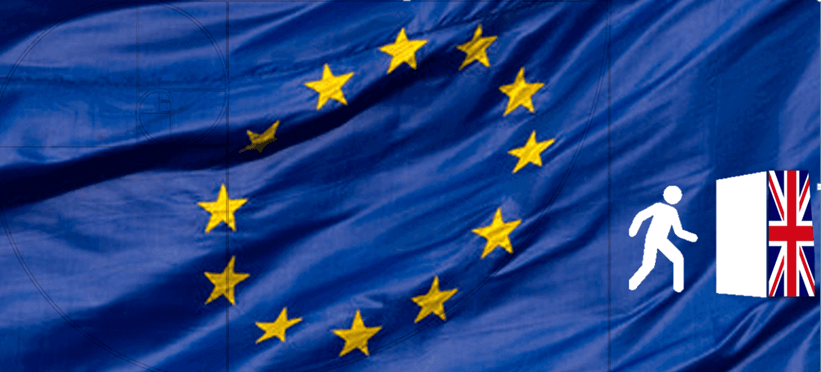# How to use Fibonacci during the Brexit week? - video21 June, AtoZForex -  It is Brexit week and "Brexit" word is flying everywhere, many of the AtoZForex followers have been asking how to use Fibonacci during the Brexit week. Thus, we took our time to look into the market applying true Fibonacci waves.

Before applying Fibonacci to the market, you need to know how to actually use Fibonacci. Although there are multiple Fibonacci strategies out there, in Yagub Rahimov's True Fibonacci Waves theory I have focused on putting the market move in a mathematically sound way, which I am covering in my upcoming "Forex NOT for dummies" trader's journey book. Based on the True Fibonacci Waves theory applying Fibonacci on highest high (hereby HH) and lowest low (hereby LL) is just like jumping in the river and trying to swim upstream. Hence, you have to go back to the basics of the Fibonacci theory applied on mathematics.  Thus, any wave that Fibonacci retracement zone is applied must be a true Fibonacci wave.

### What is a true fibonacci wave?

A true fibonacci wave is a market movement made of certain number of candlesticks which are uninterrupted and are made of fibonacci numbers.  As an example, if you want to draw your fibonacci wave on a bearish trend, you need to make sure that all the candles you have in your specified wave are bearish, with no bullish candle in between.

The next True Fibonacci wave requirement is based on the number of candles (periods), hence your wave must be made of fibonacci numbers (1, 2, 3, 5, 8, 13 etc...). If the wave is imperfect, your result won't be perfect neither. As an example of this analysis you can see my GBPUSD analysis posted on March 21 2016, where we mentioned that GBPUSD will be bouncing off the 1.4675 and become bearish until 1.3990 before going bullish again. This is a total market movement of over 1.000 pips. Watch the video analysis here.

There are more requirements for the true Fibonacci wave strategy, which is outlined in the first 5 minutes of the Fibonacci video demonstration above.

### How to use Fibonacci during the Brexit week?

Now, back to the golden question: - How to use Fibonacci during the Brexit week?

To trade Brexit, one must first understand the fundamentals as a priority. Since Brexit poses a risk against the stability of the EU, there will be opportunities in Gold, CHF, USD, JPY and AUD, see the video analysis for more information on how to take advantage of this opportunity.

Tip: Focus on the section where we have commented that the best trade opportunity is on Monday the 27th of June.